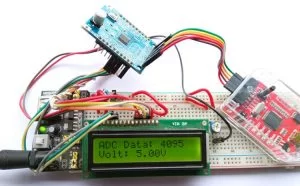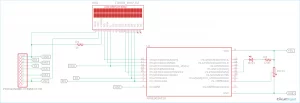# How to Use Nuvoton N76E003 Microcontroller ADC to Read Analog Voltage

Analog to Digital converter (ADC) is the most used hardware feature on a microcontroller. It takes in analog voltage and converts it to a digital value. Since microcontrollers are digital devices and work with the binary digit 1 and 0, it could not process the analog data directly. Thus, an ADC is used to take in analog voltage and convert it into its equivalent digital value that a microcontroller can understand. If you want more about Analog to Digital Converter (ADC), you can check the article linked.There are different sensors available in electronics that provide Analog output, like the MQ gas sensors, ADXL335 Accelerometer sensor, etc. Thus, using Analog to Digital converter, those sensors can be interfaced with a microcontroller unit. You can also check out other tutorials listed below, for using ADC with other microcontrollers.

In this tutorial, we will use the inbuilt ADC peripheral of the N76E003 microcontroller unit so let’s evaluate what kind of hardware setup we require for this application.

### Components Required and Hardware Setup

To use ADC on N76E003, we will use a voltage divider using a potentiometer and read the voltage ranging from 0V-5.0V. The Voltage will be displayed in the 16×2 Character LCD, if you are new with LCD and N76E003, you can check how to interface LCD with Nuvoton N76E003. Thus, the major component that is required for this project is 16×2 Character LCD. For this project, we will use the below components-

1. Character LCD 16×2
2. 1k resistor
3. 50k potentiometer or trim pot
4. Few Berg wires
5. Few hookup wires

Not to mention, other than the above components, we need the N76E003 microcontroller-based development board as well as the Nu-Link Programmer. An additional 5V power supply unit is also required as the LCD draws sufficient current that the programmer could not provide.

### Nuvoton N76E003 Circuit Diagram to Read Analog VoltageAs we can see in the schematic, the port P0 is used for the LCD related connection. On the extreme left, the programming interface connection is shown. The potentiometer acts as a voltage divider and that is sensed by the analog input 0 (AN0).

### Information about GPIO and Analog Pins in N76E003

The below image is illustrating the GPIO pins available on the N76E003AT20 microcontroller unit. However, out of the 20 pins, For the LCD related connection, the Port P0 (P0.0, P0.1, P0.2, P0.4, P0.5, P0.6, and P0.7) is used. The Analog pins are highlighted in RED colors.

As we can see, the Port P0 has maximum analog pins but those are used for LCD related communication. Thus, P3.0 and P1.7 are available as Analog input pins AIN1 and AIN0. As this project requires only one analog pin, P1.7 which is Analog input channel 0, is used for this project.

N76E003 provides a 12-bit SAR ADC. It is a very good feature of the N76E003 that it has a very good resolution of ADC. The ADC has 8-Channel inputs in single-end mode. Interfacing the ADC is pretty simple and straightforward.

The first step is to select the ADC channel input. There are 8-Channel inputs available in N76E003 microcontrollers. After selecting the ADC inputs or the I/O pins, all the pins are required to be set for the direction in the code. All the pins used for the Analog input are input pins of the microcontroller thus all the pins need to be set as Input-only (high-impedance) mode. These can be set using the PxM1 and PxM2 register. These two registers set the I/O modes where the x stands for the Port number (For example, Port P1.0 the register will be P1M1 and P1M2, for P3.0 it will be P3M1 and P3M2, etc.) The configuration can be seen in the below image-

The first 4 bits of the register from bit 0 to bit 3 is used to set the ADC Channel selection. Since we are using the channel AIN0, the selection will be 0000 for these four bits.

The 6th and 7th bits are the important ones. ADCS is required to set 1 for starting the ADC conversion and the ADCF will provide information about the successful ADC conversion. It needs to be set 0 by the firmware for starting the ADC conversion. The next register is the ADCCON1-

The ADCCON1 register is mainly used for the ADC conversion triggered by external sources. However, for normal polling related operations, the first-bit ADCEN is required to set 1 for turning on the ADC circuitry.

Next, the input of the ADC channel needs to be controlled in the AINDIDS register where the digital inputs can be disconnected.